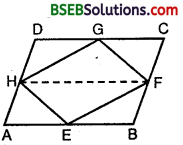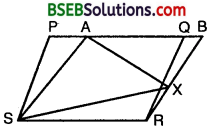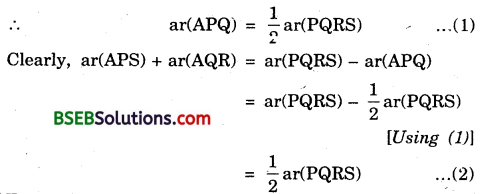# Bihar Board Class 9th Maths Solutions Chapter 9 Areas of Parallelograms and Triangles Ex 9.2

Bihar Board Class 9th Maths Solutions Chapter 9 Areas of Parallelograms and Triangles Ex 9.2 Textbook Questions and Answers.

## BSEB Bihar Board Class 9th Maths Solutions Chapter 9 Areas of Parallelograms and Triangles Ex 9.2Question 1.
In figure, ABCD is a parallelogram, AE ⊥ DC and CF ⊥ AD. If AB = 16 cm, AE = 8 cm and CF = 10 cm, find AD.Solution:
We have,
Area of a ||gm = Base x Height
∴ Area of ||gm ABCD = AB x AE
= (16 x 8) cm² = 128 cm² … (1)
Also, Area of ||gm ABCD = AD x CF
I = (AD x 10) cm² … (2)
From (1) and (2), we get
⇒ AD = $$\frac { 128 }{ 10 }$$ cm = 12.8 cm.Question 2.
If E, F, G and H are respectively the mid-points of the sides of a parallelogram ABCD, show that ar (EFGH) = $$\frac { 1 }{ 2 }$$ar(ABCD).
Solution:
A HGF and ||gm HDCF stand on the same base HF and lie between the same parallels HF and DC.∴ ar(HEF) = $$\frac { 1 }{ 2 }$$ ar(ABFH) … (1)
Similarly, ∆ HEF and ||gm ABFH stand on the same base HF and lie between the same parallels HF and AB.
∴ ar(HEF) = $$\frac { 1 }{ 2 }$$ ar(ABFH) … (2)
∴ Adding (1) and (2), we get
ar(HGF) + ar(∆ HEF) = $$\frac { 1 }{ 2 }$$ [ar(HDCF) + ar(ABFH)]
⇒ ar(EFGH) = $$\frac { 1 }{ 2 }$$ ar(ABCD).Question 3.
P and Q are any two points lying on the sides DC and AD respectively of a parallelogram ABCD. Show that ar(APB) = ar(BQC).
Solution:
∆ APB and ||gm ABCD stand on the same base AB and lie between the same parallels AB and DC.∴ ar(APB) = $$\frac { 1 }{ 2 }$$ ar(ABCD) … (1)
Similarly, ∆ BQC and ||gm ABCD stand on the same base BO and lie between the same parallels BC and AD.
∴ ar(BQC) = $$\frac { 1 }{ 2 }$$ ar(ABCD)
From (1) and (2), we have
ar(APB) = ar(BQC).Question 4.
In figure, P is a point in the interior of a parallelogram ABCD. Show that
(i) ar(APB) + ar(PCD) = $$\frac { 1 }{ 2 }$$ ar(ABCD)
(ii) ar(APD) + ar(PBC) = ar(APB) + ar(PCD).Solution:
Draw EPF parallel to AB or DC and GPH parallel to AD or BC.Now AGHD is a ||gm [∵ GH || DA and AG || DH]
Similarly, HCBG, EFCD and ABFE are parallelograms.
(i) ∆ APB and ||gm ABFE stand on the same base AB and lie between the same parallels AB and DC.
∴ ar(APB) = $$\frac { 1 }{ 2 }$$ ar(ABFE) … (1)
Similarly, ar(PCD) = $$\frac { 1 }{ 2 }$$ ar(EFCD)
Adding (1) and (2), we get
ar(APB) + ar(PCD) = $$\frac { 1 }{ 2 }$$ [ar(ABFE) + ar(EFCD)]
= $$\frac { 1 }{ 2 }$$ ar(ABCD) … (3)

(ii) ∆ APD and ||gm AGHD are on the same base AD and lie between the same parallels AD and HG.
∴ ar(APD) = $$\frac { 1 }{ 2 }$$ ar(AGHD) … (4)
Similarly, ar(PCB) = $$\frac { 1 }{ 2 }$$ar(GBCH) … (5)
Adding (4) and (5), we get
ar(APD) + ar(PCB) = $$\frac { 1 }{ 2 }$$ [ar(AGHD) + ar(GBCH)]
= $$\frac { 1 }{ 2 }$$ ar(ABCD) … (6)
From (3) and (6), we get
ar(APD) + ar(PBC) = ar(APB) + ar(PCD).Question 5.
In figure, PQRS and ABRS are parallelograms and X is any point on side BR. Show that
(i) ar(PQRS) = $$\frac { 1 }{ 2 }$$ ar(ABJtS)
(ii) ar(AXS) = $$\frac { 1 }{ 2 }$$ ar(PQRS).Solution:
(i) ||gm PQRS and ||gm ABRS stand on the same base RS and lie between the same parallels SR and PAQB.
∴ ar(PQRS) = ar(ABRS) … (1)

(ii) ∆ AXS and ||gm ABRS stand on the same base AS and lie between the same parallels AS and RB.
∴ ar(AXS) = $$\frac { 1 }{ 2 }$$ ar(ABRS) z
ar(AXS) = $$\frac { 1 }{ 2 }$$ar(PQRS) [Using (1)]Question 6.
A farmer was having a field in the form of a parallelogram PQRS. She took any point A on RS and joined it to points P and Q. In how many parts the fields is divided? What are the shapes of these parts? The farmer wants to sow wheat and pulses in equal portions of the field separately. How should she do it?
Solution:
Clearly, the field i.e., ||gm PQRS is divided into 3 parts.Each part is of the shape of triangle.
Since ∆ APQ and ||gm PQRS stand on the same base PQ and lie between the same parallels PQ and SR.From (1) and (2), we get
ar(APS) + ar(AQR) = ar(APQ).
Thus the farmer should sow wheat and pulses either as [(∆s APS and AQR) or ∆ APQ] or as [∆ APQ or (∆s APS and AQR].